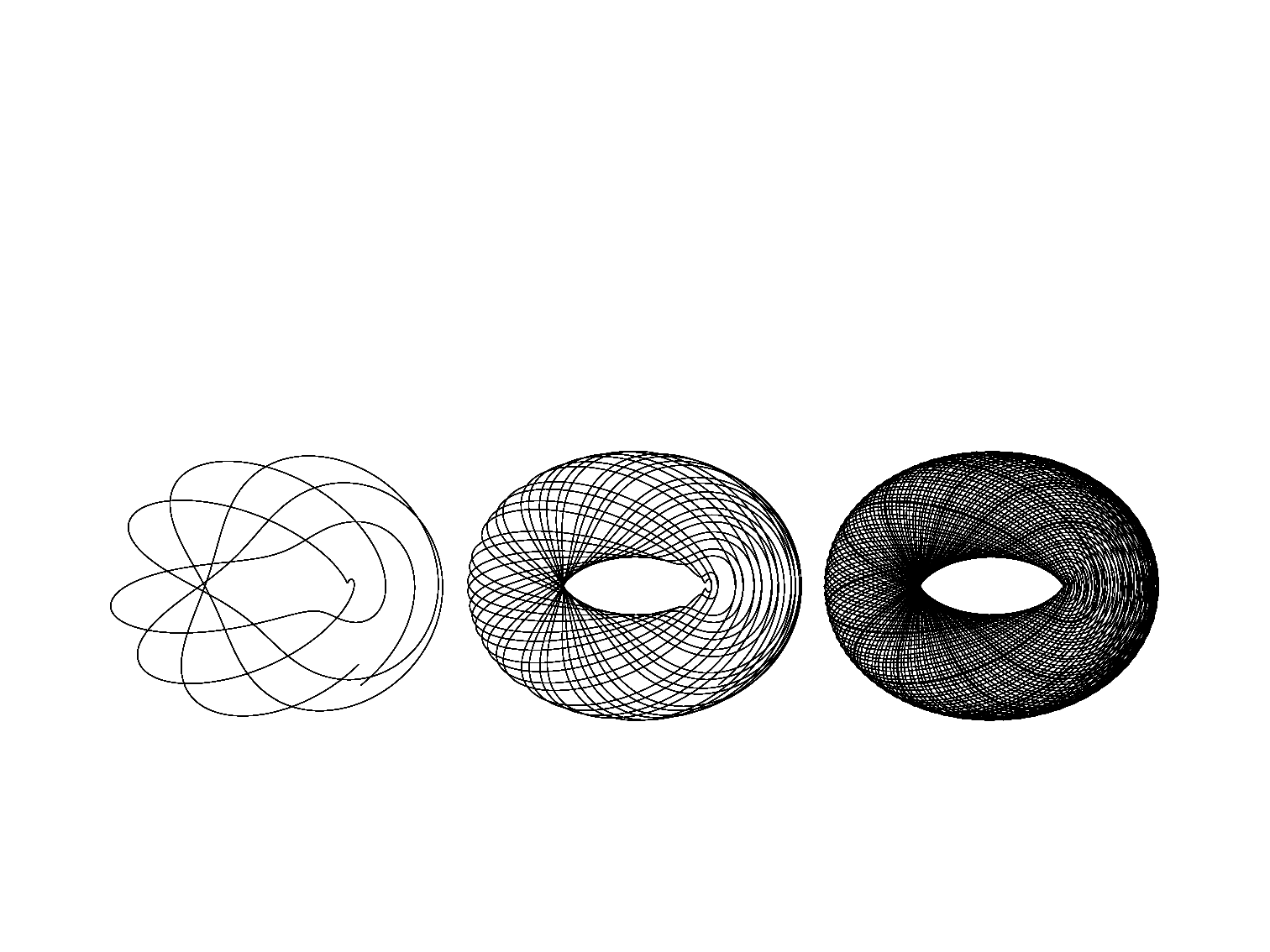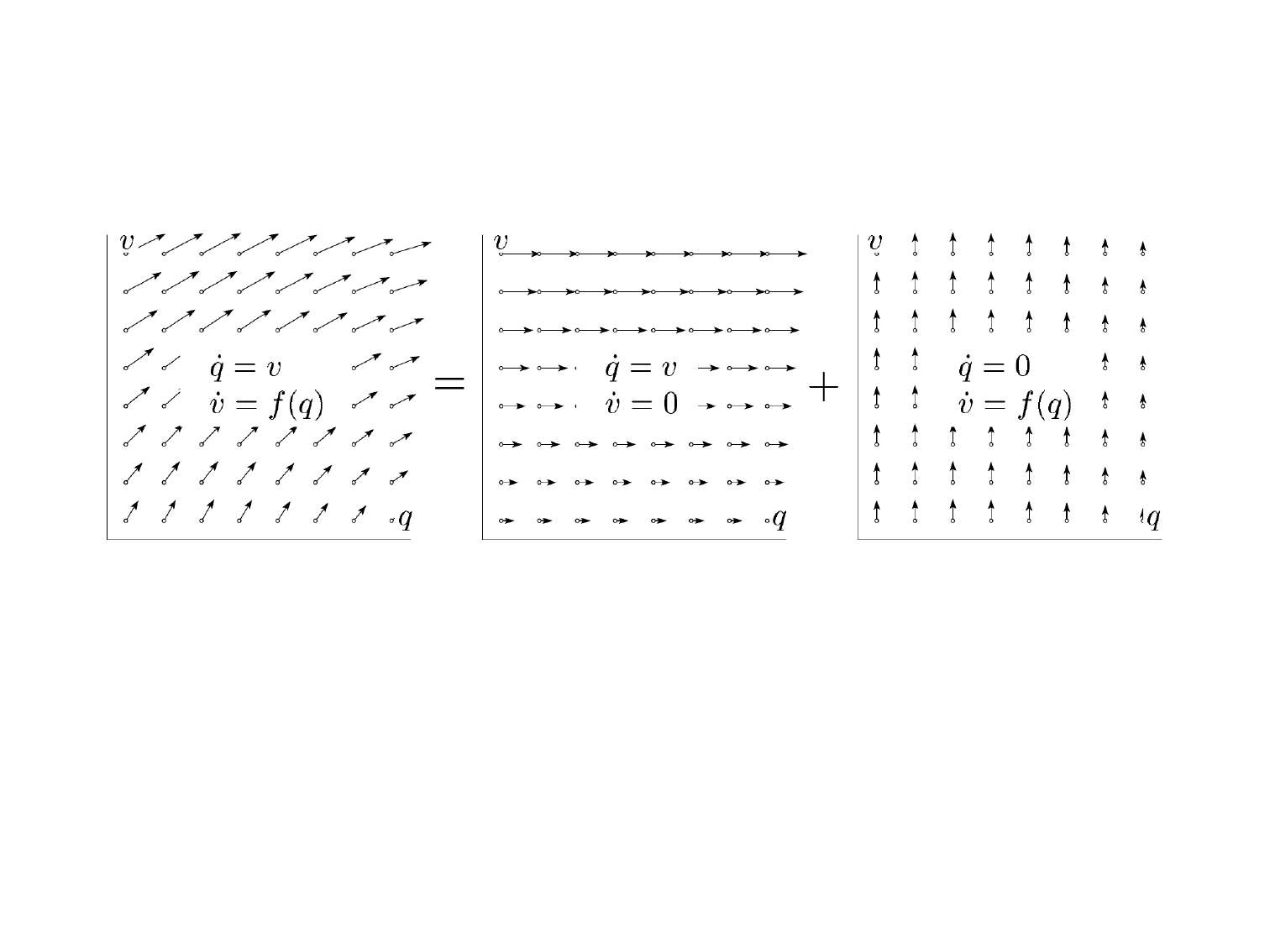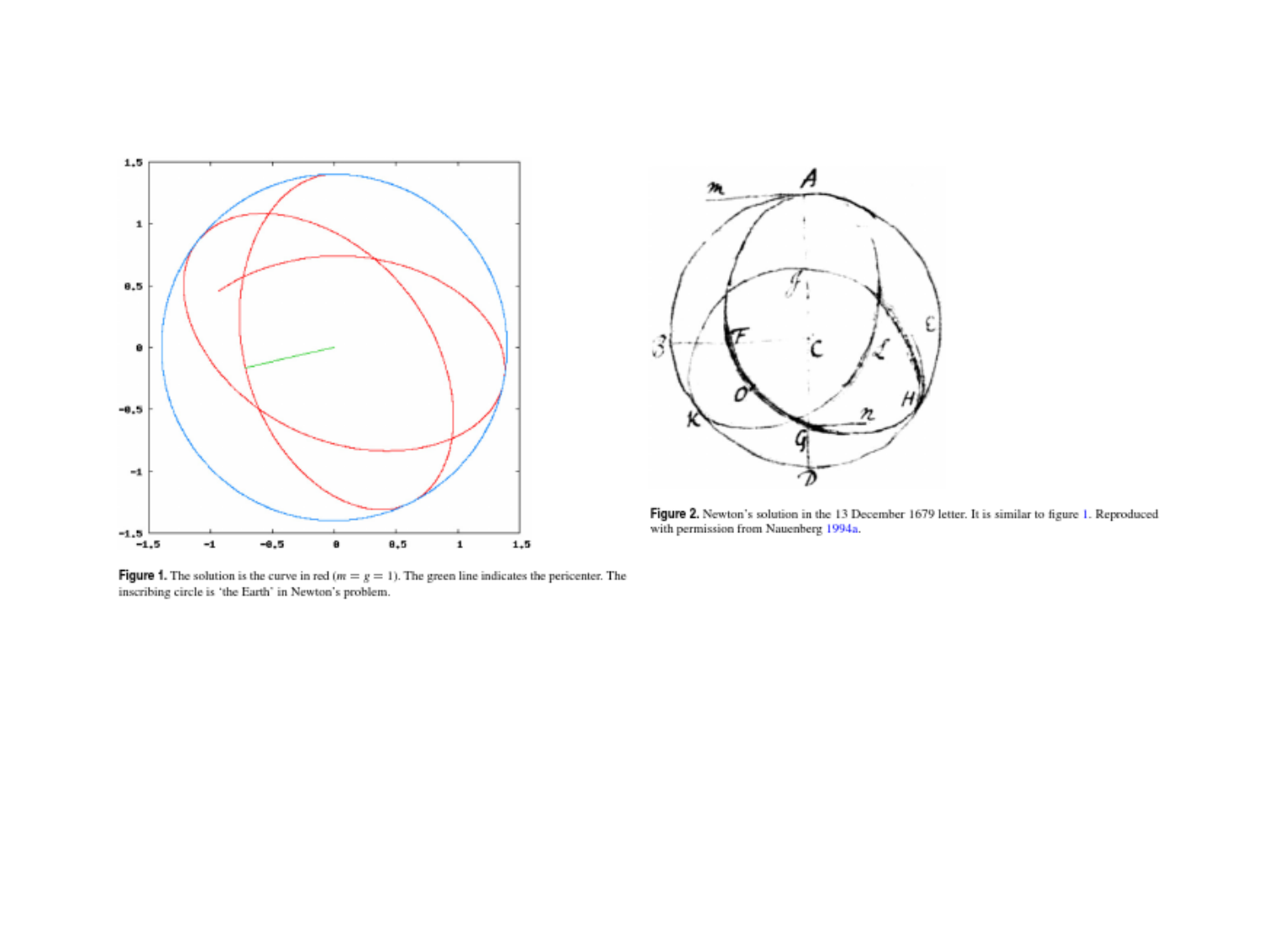Sampling the phase space of a mechanical system
Juan M. Bello-Rivas
jmb@ices.utexas.eduOutlineOur setting
Consider a system of N particles with Hamiltonian function
H : Γ R
(q, p) 7→ H(q, p),
where Γ R
6N
is the phase space of the system.
Let X = {(q, p) Γ | H(q, p) = E} for some E R. We want to
compute integrals of the form
Z
X
f(q, p) π(q, p) dq dp,
where
π(q, p) =
δ(H(q, p) E)
Ω(E)
and Ω(E) =
Z
X
δ(H(q, p)E) dq dp.Possible methods
We have already discussed two ways to do this:
1 Metropolis sampling.
2 Some analogue of what we did with the torus.Possible methods
It is hard to use Metropolis because, even though (q, p) X , we
rarely will ﬁnd that (q + q, p + p) X . That is,
H(q + q, p + p) will lie outside the level set E of H.
p
q
H(q, p) = EPossible methods
By contrast, for the torus we had a rule ϕ
t
(x) that would give us a
time-evolution which in turn would yield uniformly-distributed
samples from the torus. Can we do something like that in our
current setting?Possible methods
Yes, if the Hamiltonian is time-independent, then the solution
t 7→ (q(t), p(t)) of the system of ODEs (known as the equations of
motion),
dq
dt
(t) =
p
H(q(t), p(t)),
dp
dt
(t) = −∇
q
H(q(t), p(t)),
q(0) = q
0
,
p(0) = p
0
.
is such that H(q(t), p(t)) = H(q
0
, p
0
) = E for all t 0.Possible methods
Observe that the solution of the equations of motion can be
thought of as a mapping
ϕ: [0, ) × X X
taking the initial condition to the value of the solution at time t.
In other words,
(q
0
, p
0
) 7→ ϕ
t
(q
0
, p
0
) = (q(t), p(t)) for all t 0.
This is analogous to the scenario we had in the case of the torus,
where ϕ
t
(x, v) = x + t v.Possible methods
Therefore, it appears that solving the equations of motion gives us
a feasible way of drawing samples from the constant energy surface
X because, if the system is ergodic, then
Z
X
f(q, p) π(q, p) dq dp = lim
T →∞
1
T
T
Z
0
f(ϕ
t
(q
0
, p
0
)) dt.Solution of the equations of motion
Most ODEs cannot be solved in explicit form (i.e., by formulas
involving combinations of arithmetic operations, trigonometric
formulas, exponentials and logarithms).
Example
The diﬀerential equation
dy
dx
= y
2
x
can only be solved in terms of special functions (Airy).Solution of the equations of motion
The focus must be shifted:
Exact solutions Approximate solutions that reproduce
qualitative properties of the exact solution.Conservation of energy
dH
dt
(q(t), p(t)) =
q
H(q(t), p(t))
dq
dt
(t) +
p
H(q(t), p(t))
dp
dt
(t).
From
dq
dt
(t) =
p
H(q(t), p(t)),
dp
dt
(t) = −∇
q
H(q(t), p(t)),
We see that
dH
dt
(q(t), p(t)) = 0,
which implies that
t 7→ H(q(t), p(t)) = E,
for all t 0.Time-reversibility
Let s(t) = t, ¯q(s) = q(t), and ¯p(s) = p(t). On one hand,
dq
dt
=
dq
ds
ds
dt
=
d¯q
ds
and
H
p
=
H
¯p
¯p
p
=
H
¯p
On the other hand,
dp
dt
=
dp
ds
ds
dt
=
d¯p
ds
and
H
q
=
H
¯q
¯q
q
=
H
¯q
Therefore, if ϕ
t
(q
0
, p
0
) is a solution curve of the equations of
motion, then so is ϕ
T t
(q
T
, p
T
).Preservation of volume
Recall that the divergence tells us how much the volume changes
locally. In Cartesian coordinates, if v = (v
1
, v
2
, v
3
), then
· v =
P
3
i=1
v
i
x
i
. Going back to
dq
dt
=
H
p
,
dp
dt
=
H
q
,
we see that the divergence of the vector ﬁeld on the right hand
side is
·
H
p
,
H
q
=
2
H
q p
2
H
p q
= 0.
Remark
Symplectic transformations ( Volume-preserving transformationsTime-stepping methods
Goal: solve the system of ODEs
dz
dt
(t) = J H(z(t)),
z(0) = z
0
,
where z(t) = (q(t), p(t)) and
J =
0 I
I 0
R
6N×6N
.
Idea: approximate by the
diﬀerence equations
z
n+1
z
n
t
= J H(z
n
),
z
0
given,
where t > 0. Or by
z
n+1
z
n
t
= J H(z
n+1
),
z
0
given,Time-stepping methods
Observe that the solution of the diﬀerence equations
z
n+1
z
n
t
= J H(z
n
),
z
0
given,
induces a numerical counterpart of ϕ
t
, namely
ψ
nt
(z
0
) = z
n
,
and ψ
nt
is expected to be close to ϕ
t
whenever nt = t. How
close are these two mappings?Time-stepping methods
Explicit Euler is really solving an externally forced system:
dq
dt
=
H
p
,
dp
dt
=
H
q
+ f(t),
where f(t) is a forcing term.Time-stepping methods
Implicit Euler is really solving a dissipative system:
dq
dt
=
H
p
,
dp
dt
=
H
q
γp,
where γ > 0 is the friction coeﬃcient.Hamiltonian splitting
Let
H
B
(q, p) = T (p) where T (p) =
1
2
p
T
M
1
p.
The corresponding equations of motion are:
dq
dt
(t) = M
1
p(t),
dp
dt
(t) = 0,
q(0) = q
0
,
p(0) = p
0
.
And their exact solution is
ϕ
t,H
B
(q
0
, p
0
) = (q
0
+ t M
1
p
0
| {z }
=q(t)
, p
0
|{z}
=p(t)
).Hamiltonian splitting
Let
H
A
(q, p) = U(q)
for U : R
3N
R s.t. F (q) = −∇U(q). The corresponding
equations of motion are:
dq
dt
(t) = 0,
dp
dt
(t) = F (q(t)),
q(0) = q
0
,
p(0) = p
0
.
And their exact solution is
ϕ
t,H
A
(q
0
, p
0
) = ( q
0
|{z}
=q(t)
, p
0
+ t F (q
0
)
| {z }
=p(t)
).Hamiltonian splitting
dq
dt
(t) = M
1
p(t),
dp
dt
(t) = 0,
q(0) = q
0
,
p(0) = p
0
.
dq
dt
(t) = 0,
dp
dt
(t) = F (q(t)),
q(0) = q
0
,
p(0) = p
0
.Hamiltonian splitting
Now consider the Hamiltonian
H(q, p) =
1
2
H
B
(q, p) + H
A
(q, p) +
1
2
H
B
(q, p)
= T (p) + U(q)
and the numerical method given by
ψ
t
= ϕ
t
2
,H
B
ϕ
t,H
A
ϕ
t
2
,H
B
.Hamiltonian splitting
Reproduced from: Hairer, E., Lubich, C., and Wanner, G. (2003).
Geometric numerical integration illustrated by the St¨ormer Verlet
method. Acta Numerica, 12, 399450.Hamiltonian splitting
We can write
ψ
t
= ϕ
t
2
,H
B
ϕ
t,H
A
ϕ
t
2
,H
B
more explicitly as
q
n+
1
2
= q
n
+
t
2
M
1
p
n
,
p
n+1
= p
n
+ t F (q
n+
1
2
),
q
n+1
= q
n+
1
2
+
t
2
M
1
p
n+1
.
Notice that this involves only one force evaluation per time-step.
Furthermore, the method is trivially time-reversible and conserves
phase-space volume.Hamiltonian splitting
Reproduced from: Dias, P. M. C., Stuchi, T. J. (2013). What can
numerical computation do for the history of science? (a study of an orbit
drawn by Newton in a letter to Hooke). European Journal of Physics,
34(6), 14651485.Questions?